# Python | dtype object length of Numpy array of strings

In this post, we are going to see the datatype of the numpy object when the underlying data is of string type. In numpy, if the underlying data type of the given object is string then the dtype of object is the length of the longest string in the array. This is so because we cannot create variable length string in numpy since numpy needs to know how much space should be allocated for string.

Problem #1 : Given a numpy array whose underlying data is of string type. Find the dtype.

Solution : We will use `numpy.dtype` attribute to check the dtype of the given object.

 `# importing the numpy library as np ` `import` `numpy as np ` ` `  `# Create a numpy array ` `arr ``=` `np.array([``'Ela'``, ``'Ed'``, ``'Brook'``, ``'Sia'``, ``'Katherine'``]) ` ` `  `# Print the array ` `print``(arr) `

Output :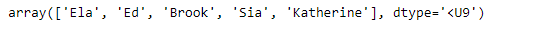Now we will check the dtype of the given array object whose underlying data is of string type.

 `# Print the dtype ` `print``(arr.dtype) `

Output :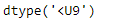As we can see in the output, the dtype of the given array object is `'<U9'` where 9 is the length of the longest string in the given array object.

Let’s verify this by checking the length of the longest string in the given object.

 `# Use vectorize function of numpy ` `length_checker ``=` `np.vectorize(``len``) ` ` `  `# Find the length of each element ` `arr_len ``=` `length_checker(arr) ` ` `  `# Print the length of each element ` `print``(arr_len) ` ` `  `# Print the maximum value ` `print``(arr_len.``max``()) `

Output :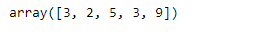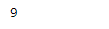Problem #2 : Given a numpy array whose underlying data is of string type. Find the dtype.

Solution : We will use `numpy.dtype` attribute to check the dtype of the given object.

 `# importing the numpy library as np ` `import` `numpy as np ` ` `  `# Create a numpy array ` `arr ``=` `np.array([``'New York'``, ``'Lisbon'``, ``'Beijing'``, ``'Quebec'``]) ` ` `  `# Print the array ` `print``(arr) `

Output :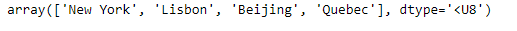Now we will check the dtype of the given array object whose underlying data is of string type.

 `# Print the dtype ` `print``(arr.dtype) `

Output :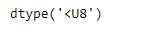As we can see in the output, the dtype of the given array object is `'<U8'` where 8 is the length of the longest string in the given array object.

Let’s verify this by checking the length of the longest string in the given object.

 `# Use vectorize function of numpy ` `length_checker ``=` `np.vectorize(``len``) ` ` `  `# Find the length of each element ` `arr_len ``=` `length_checker(arr) ` ` `  `# Print the length of each element ` `print``(arr_len) ` ` `  `# Print the maximum value ` `print``(arr_len.``max``()) `

Output :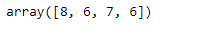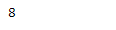My Personal Notes arrow_drop_upCheck out this Author's contributed articles.

If you like GeeksforGeeks and would like to contribute, you can also write an article using contribute.geeksforgeeks.org or mail your article to contribute@geeksforgeeks.org. See your article appearing on the GeeksforGeeks main page and help other Geeks.

Please Improve this article if you find anything incorrect by clicking on the "Improve Article" button below.

Article Tags :

Be the First to upvote.

Please write to us at contribute@geeksforgeeks.org to report any issue with the above content.